# Useful Kinect Info

It took too long surfing for this information, and its still scattered across the internet.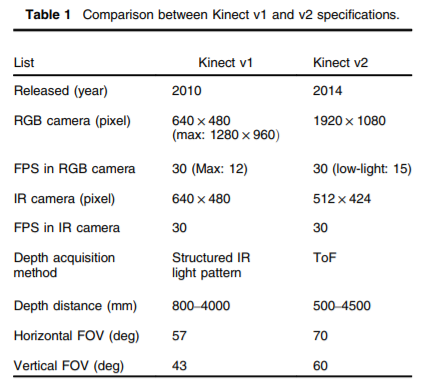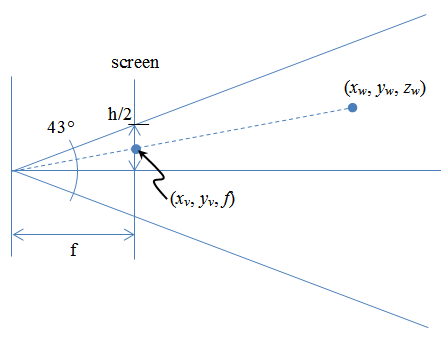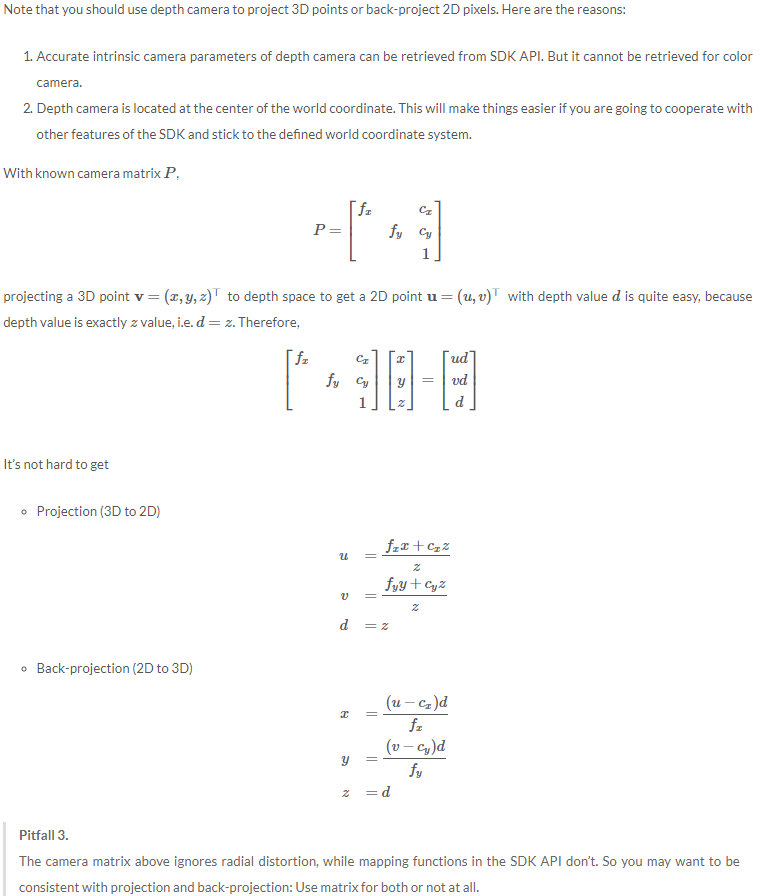The projection matirix is used to convert from 3D read world coordintes to 2D image coordinates. The structure of this projection matrix is shown in figure 2. We use linear regression to estimate the elements of the 3x4 matrix generated as a product of intrinsic and extrinsic properties of the image.#### From spherical coordinates

{\begin{aligned}x&=\rho \,\sin \theta \,\cos \phi \\y&=\rho \,\sin \theta \,\sin \phi \\z&=\rho \,\cos \theta \\{\frac {\partial (x,y,z)}{\partial (\rho ,\theta ,\phi )}}&={\begin{pmatrix}\sin \theta \cos \phi &\rho \cos \theta \cos \phi &-\rho \sin \theta \sin \phi \\\sin \theta \sin \phi &\rho \cos \theta \sin \phi &\rho \sin \theta \cos \phi \\\cos \theta &-\rho \sin \theta &0\end{pmatrix}}\end{aligned}}References :

## Comment viewing options### Polar to Cartesian - find

Any ideas what is wrong with this logic ? The result in JMonkey looks like crap.

Polar to Cartesian - find theta and phi

To find theta & phi you need focal length

kinect vertical focal length - 43
kinect horizontal focal length - 57

we want theta in radians / pixel

kinect vertical is  (43 * 0.0174533)/480  = 0.0015635
kinect horizontal is (57 * 0.0174533)/640 = 0.0015544

zw = depth * Math.cos(yv * verticalRadiansPerPixel)
yw = depth * Math.sin(yv * verticalRadiansPerPixel)
xw = depth * Math.sin(xv * horizontalRadiansPerPixel)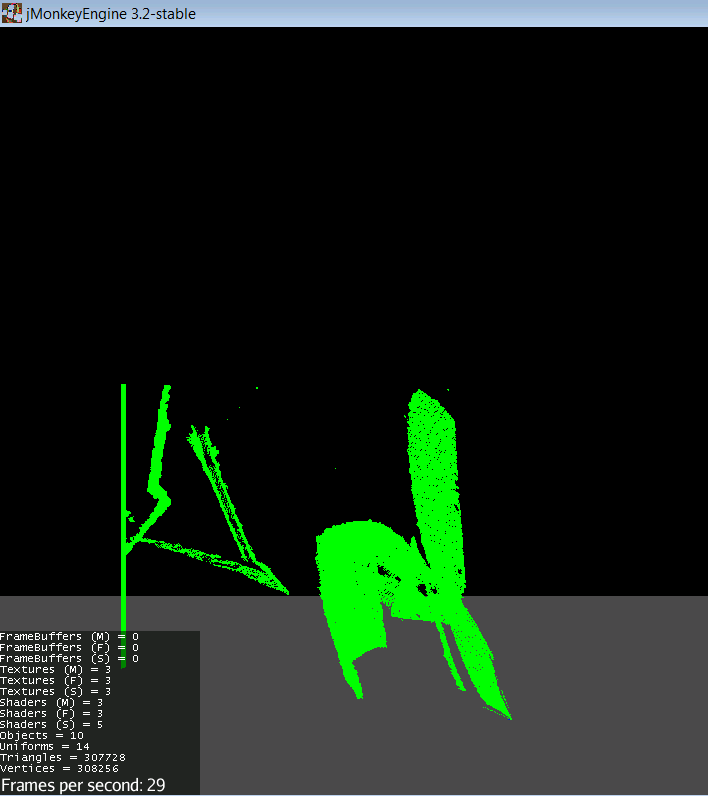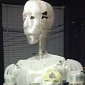### spherical is good for projection...

So., the way i see it, you want to go from the screen coordinate system to the world coordinate system.

Screen coordinates are defined as an x and y position on the screen at a specific focal length f.

so.. you can go from xv,yv,fv  to spherical coordinates.

Once you ahve the spherical coordinates for that dot on the screen.. add the depth from the depth map for that pixel to the radium.

So, the screen spherical coordinates are

r(v), theta(v), phi(v)

.. now that we're in spherical coordinates, we can add the "depth" from the depth map at that pixel location to the radius to project our point into real world coordinates.  (notice in this geometry, theta and phi are the same for both points as they are in a straight line with respect to the origin of the spherical coordinate system.)

r(v) + depth , theta(v), and phi(v)

Take the coordinate system x-form from spherical to carteasian ..and presto you have

Xw, Yx, Zw,   (with respect to the origin of the camera.)

Now, if you know the position and orientation of the camera, you can create a homogenous transformation matrix (translate+rotate) to align the camera with some other coordinate system if desired.